OPTIMISATION PROBLEMS Analysis

We are often only interested in either the maximum or minimum of a function. In order to solve these kinds of problem you should do the following:

1. Determine from which function you want to find out the maximum or minimum. The function could easily have more than one independent variable; if this is the case then find the relation between them so that you are left with just one unknown in the function.

2. Find the maximum or minimum you are looking for by applying the necessary conditions to their derivatives.

3. Evaluate the solution you obtain

Let's look at a couple of examples.

EXAMPLE 1

A company has decided to improve its security by installing 9 alarms. A specialist points out to the company that given the kind of company that it is they can only opt for two types of alarms: type A or type B. Furthermore, he states that the company's security can be expressed as a tenth of the product of the number of type A alarms multiplied by the square of the number of type B alarms. How many of each type of alarm should the company have installed in order to maximise their security?

a. Determine the function
• Let x be the number of type B alarms installed and (9-x) be the number of type A alarms.

• The company's security is expressed by the function f(x)=(9-x)x2/10=(9x2-x3)/10

b. Find the maximum

• We get f'(x)=(18x-3x2)/10

• We solve the equation: f'(x)=0. Solutions: x=0, x=6

• We get f''(x)=(18-6x)/10 and find its sign for these values. The maximum is found at x=6

c. Evaluate the solution

• We should install 6 type B alarms and 3 type A alarms

 The graphs of f' and f'' appear in the window. Change the value of x to check your results.

EXAMPLE 2

There is a power station on the banks of a 1 km wide river. On the other side of the river there is a factory 8 km upstream. It costs 0.30/metre to run a cable on land and 0.50/metre to run it underwater. What is the cheapest way of running cable from the power station to the factory.

 Look at the graph in the window. C and F show the situation of the Power Station (C) and the Factory (F). Click on the blue arrow to alter the value of x. Point P is where the cable starts to run underwater. Keep changing the value of x and look carefully at the results.

a. Determine the function

• Cost: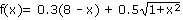thousand euros.

b. Find the minimum

• Find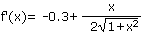• Solve the equation: f'(x)=0. Solutions: x=0.75 and x=-0.75 (Note that the negative solution is irrelevant here)

• Find f''(x) and its sign when x=0.75 and check that this is a minimum.

c. Evaluate the solutions

• The cheapest option is to run the cable under the river 7.25 km away from the power station, or from the factory, due to symmetry. This gives us the minimum cost of 2,800.

You can now do some exercises:                 EXERCISE 1        EXERCISE 2        EXERCISE 3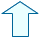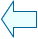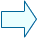María José García CebrianSpanish Ministry of Education. Year 2001